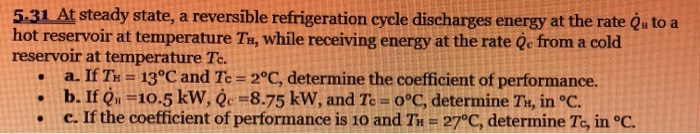# Question 5.31 At steady state, a reversible refrigeration cycle discharges energy at the rate Ou to a hot reservoir at temperature Ty, while receiving energy at the rate Qc from a cold reservoir at temperature Tc. a. If Tk = 13°C and Tc=2°C, determine the coefficient of performance. b. If (x =10.5 kW, èc =8.75 kW, and Te = 0°C, determine Tv, in °C. c. If the coefficient of performance is 10 and TH = 27°C, determine T., in °C.VHBKKA The Asker · Mechanical EngineeringTranscribed Image Text: 5.31 At steady state, a reversible refrigeration cycle discharges energy at the rate Ou to a hot reservoir at temperature Ty, while receiving energy at the rate Qc from a cold reservoir at temperature Tc. a. If Tk = 13°C and Tc=2°C, determine the coefficient of performance. b. If (x =10.5 kW, èc =8.75 kW, and Te = 0°C, determine Tv, in °C. c. If the coefficient of performance is 10 and TH = 27°C, determine T., in °C.
More
Transcribed Image Text: 5.31 At steady state, a reversible refrigeration cycle discharges energy at the rate Ou to a hot reservoir at temperature Ty, while receiving energy at the rate Qc from a cold reservoir at temperature Tc. a. If Tk = 13°C and Tc=2°C, determine the coefficient of performance. b. If (x =10.5 kW, èc =8.75 kW, and Te = 0°C, determine Tv, in °C. c. If the coefficient of performance is 10 and TH = 27°C, determine T., in °C.